• 设计判断二叉树是否为二叉排序树的算法 bool isSortTree(TreeNode *Tree) 递归判断二叉树是否为二叉排序树， 1.1 叶子结点返回true，即N0结点 1.2 只有左右子树的其中一个，N1结点 1.3 有左右子树的，N2结点 二叉...

设计判断二叉树是否为二叉排序树的算法

1. bool isSortTree(TreeNode *Tree) 递归判断二叉树是否为二叉排序树，
1.1 叶子结点返回true，即N0结点
1.2 只有左右子树的其中一个，N1结点
1.3 有左右子树的，N2结点
2. 二叉排序树性质：中序遍历二叉树 得到的序列值是递增的。
// 1、设计判断二叉树是否为二叉排序树的算法。（8分）
#include "stdio.h"
#include "stdbool.h"
#include "stdlib.h"

typedef struct TreeNode
{
int data;               //数据域
TreeNode *left, *right; //指向其左右孩子结点
} TreeNode;

bool isSortTree(TreeNode *Tree);

void showTree(TreeNode *Tree);
int main(int argc, char const *argv[])
{
TreeNode Node4 = {.data = 7, .left = NULL, .right = NULL};
TreeNode Node5 = {.data = 9, .left = NULL, .right = NULL};
TreeNode Node6 = {.data = 11, .left = NULL, .right = NULL};
TreeNode Node7 = {.data = 13, .left = NULL, .right = NULL};

TreeNode Node2 = {.data = 8, .left = &Node4, .right = &Node5};
// TreeNode Node2 = {.data = 8, .left = &Node5, .right = &Node4}; // No
// TreeNode Node2 = {.data = 8, .left = NULL, .right = NULL}; // Yes

TreeNode Node3 = {.data = 12, .left = &Node6, .right = &Node7};
TreeNode Node1 = {.data = 10, .left = &Node2, .right = &Node3};

showTree(&Node1);
bool res = isSortTree(&Node1);
printf("\n%s", res ? "yes" : "No");
return 0;
}

bool isSortTree(TreeNode *Tree)
{
if (!Tree)
return true;
if (!Tree->left && !Tree->right)
{
return true;
}

if (Tree->left && !Tree->right)
{
if (Tree->data > Tree->left->data)
return true;
else
return false;
}

if (Tree->right && !Tree->left)
{
if (Tree->data < Tree->right->data)
return true;
else
return false;
}

if (Tree->data > Tree->left->data && Tree->data < Tree->right->data)
{

bool ll = isSortTree(Tree->left);
bool rr = isSortTree(Tree->right);
if (ll && rr)
{
return true;
}
else
{
return false;
}
}
else
{
return false;
}
}

void showTree(TreeNode *Tree)
{
if (Tree)
{
showTree(Tree->left);
printf("%d ,", Tree->data);
showTree(Tree->right);
}
}

result:

7 ,8 ,9 ,10 ,11 ,12 ,13 ,
yes展开全文• 题目：编写一个算法判断给定的二叉树是否二叉排序树 分析： 二叉排序树的中序序列是升序序列，我们可以根据这一特性来进行判定 typedef struct node { int data; node *left, *right; }Tree; #define _CRT...

题目：编写一个算法判断给定的二叉树是否是二叉排序树

分析：
二叉排序树的中序序列是升序序列，我们可以根据这一特性来进行判定

typedef struct node {
int data;
node *left, *right;
}Tree;
#define _CRT_SECURE_NO_WARNINGS
#include <stdio.h>
#include <stdlib.h>
Tree *create(Tree *T) {//先序创建一颗二叉树
int data;
printf("请输入当前节点值：data=");
scanf("%d", &data);
getchar();
if (data != -1) {
T = (Tree *)malloc(sizeof(Tree));
T->data = data;
T->left = NULL;
T->right = NULL;
T->left = create(T->left);
T->right = create(T->right);
}
return T;
}
bool isSortTree(Tree *T) {
static int min = -32768;//最开始设定min为最小值，确保第一个节点能够进行下去
static bool flag = true;//作为是否是排序的标记，采用静态变量，不然每次都会初始化
if (T) {
isSortTree(T->left);
if (T->data < min)
flag = false;
else
min = T->data;
isSortTree(T->right);
}
return flag;
}
int main() {
//先创建一颗二叉树
Tree *T = (Tree *)malloc(sizeof(Tree *));
T = create(T);
isSortTree(T) ? printf("是二叉排序树") : printf("不是二叉排序树");
return 0;
}

人的一生中不可能会一帆风顺，总会遇到一些挫折，当你对生活失去了信心的时候，仔细的看一看、好好回想一下你所遇到的最美好的事情吧，那会让你感觉到生活的美好。

展开全文算法 数据结构 二叉树 二叉排序树
• 判断一棵树是否为二叉排序树 思路 二叉排序树的中序遍历是有序的，所以判断一棵排序树可以进行中序遍历并且在遍历过程中判断是否有序。 为此设置一个全局变量记录上一个中序遍历节点的值，遍历过程中与全局变量比...

判断一棵树是否为二叉排序树

思路

• 二叉排序树的中序遍历是有序的，所以判断一棵排序树可以进行中序遍历并且在遍历过程中判断是否有序。
• 为此设置一个全局变量记录上一个中序遍历节点的值，遍历过程中与全局变量比大小并更新全局变量的值。

实现

#include<stdio.h>

typedef struct node
{
int data;
struct node *lchild;
struct node *rchild;
}BTNode;

int num=-32767;	//定义最小的全局变量

//中序遍历思想，并且更新全局变量的值
int Judge(BTNode *&p)
{
int i;
if(p==NULL) return 1;	//空节点则返回

i=Judge(p->lchild);		//遍历并判断左子树是否满足二叉排序
if(i==0) return 0;

if(p->data>num)		//如果符合二叉排序树，更新全局变量的值
num=p->data;
else
return 0;

return  Judge(p->rchild);//最后判断右子树是否满足
}

展开全文数据结构 二叉树
• 今天的题目并不很难，主要想把二叉排序树实现下，也方便我以后复习使用。 二叉排序树 定义 二叉排序树（BST），也叫二叉查找树。二叉排序树或者是一颗空树， 或者是一颗具有下列特性的非空二叉树： 1.若左子树...

今天运动会开幕式结束！国旗班顺利收关！这一阵学习落下很多，以后得花很多时间在学习上了。但也很开心圆满完成国旗班任务。
今天的题目并不很难，主要想把二叉排序树实现下，也方便我以后复习使用。

二叉排序树

定义

二叉排序树（BST），也叫二叉查找树。二叉排序树或者是一颗空树，
或者是一颗具有下列特性的非空二叉树：

1.若左子树非空，则左子树上所有结点关键字值均小于根结点的关键字值

2.若右子树非空，则右子树上所有结点关键字值均大于根结点的关键字值

3.左、右子树本身也分别是一颗二叉排序树。
对二叉排序树进行中序遍历，可以得到一个递增的有序序列。

例：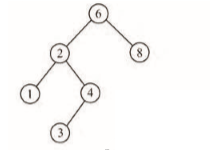操作

定义

typedef struct BinTreeNode
{
int data;
struct BinTreeNode *Lchild;
struct BinTreeNode *Rchild;
}BinTreeNode,*BinTree;

建立

注意此处要用两个函数（输入-1结束）

void InsertBinTree(BinTreeNode **T,int elem)//插入二叉排序树结点（因为要从上往下一步一步找，所以一次只能插一个，不能递归）
{
if(*T==NULL)
{
*T=(BinTreeNode*)malloc(sizeof(BinTreeNode));
(*T)->data=elem;
(*T)->Lchild=NULL;
(*T)->Rchild=NULL;
}
else if(elem<(*T)->data)
InsertBinTree(&((*T)->Lchild),elem);
else if(elem>(*T)->data)
InsertBinTree(&((*T)->Rchild),elem);

}

void CreateBinTree(BinTreeNode **T)
{
int elem;
(*T)=NULL;
scanf("%d",&elem);
while(elem!=-1)
{
InsertBinTree(T,elem);
scanf("%d",&elem);
}
}

查找

查找单个元素

BinTreeNode* SelectTree(BinTreeNode *T,int elem)//查找二叉排序树中的元素
{
if(T==NULL) return NULL;
else if(T->data==elem) return T;
else if(T->data>elem) return (SelectTree(T->Lchild,elem));
else return (SelectTree(T->Rchild,elem));

查找范围内元素

void SelectRangeelem(BinTreeNode *T,int a,int b)
{
if(T)
{
SelectRangeelem(T->Lchild,a,b);
if(T->data>a&&T->data<b) printf("%d ",T->data);
SelectRangeelem(T->Rchild,a,b);
}
}

删除

void DelBST(BinTreeNode *T,int key)//在二叉排序树中删除结点
{//因为一个结点一旦有左子树，就要有区分左右大小的任务，所以以有无左子树来分类讨论
BinTreeNode *p,*f,*s,*q;
p=T;
f=NULL;
while(p)//查找为key的待删除结点
{
if(p->data==key) break;//找到则break
f=p;//f指向p的双亲结点
if(p->data>key) p=p->Lchild;
else p=p->Rchild;
}
if(p==NULL) return ;//找不到则退出
if(p->Lchild==NULL)//若p无左子树
{
if(f==NULL) free(p);//p为原树的根
else if(f->Lchild==p)//p为f的左子树
f->Lchild=p->Rchild;//把p的右子树链到f的左子树上
else//p为f的右子树
f->Rchild=p->Rchild;//把p的右子树链到f的右子树上
free(p);
}
else//p有左子树
{
q=p;
s=q->Lchild;
while(s->Rchild)
{
q=s;
s=s->Rchild;//在p的左子树中查找最右下结点（此节点为首个比待删除结点小的结点，它能肩负起区分大小的任务）
}
if(q==p) q->Lchild=s->Lchild;
else q->Rchild=s->Lchild;//把s的左子树链到s的双亲结点p的右子树（绕开s）
p->data=s->data;
free(s);
}

}

判断是否为二叉排序树

int SelectBinSortTree(BinTreeNode *T)//判断是否为二叉排序树
{
if(T==0) return 1;
else if(T->Lchild&&T->Rchild)
{
if(T->data<T->Lchild->data||T->data>T->Rchild->data) return 0;
else return(SelectBinSortTree(T->Lchild)&&SelectBinSortTree(T->Rchild));
}
else if(T->Lchild)
{
if(T->data<T->Lchild->data) return 0;
else return(SelectBinSortTree(T->Lchild));
}
else if(T->Rchild)
{
if(T->data<T->Rchild->data) return 0;
else return(SelectBinSortTree(T->Rchild));
}
return 1;
}

总体测试代码如下

#include<stdio.h>
#include<stdlib.h>

typedef struct BinTreeNode
{
int data;
struct BinTreeNode *Lchild;
struct BinTreeNode *Rchild;
}BinTreeNode,*BinTree;

void CreateTree(BinTreeNode **T)//建立二叉树
{
int elem;
scanf("%d",&elem);
if(elem==-1) *T=NULL;
else{
*T=(BinTreeNode*)malloc(sizeof(BinTreeNode));
(*T)->data=elem;
CreateTree(&((*T)->Lchild));
CreateTree(&((*T)->Rchild));
}
}

void InsertBinTree(BinTreeNode **T,int elem)//插入二叉排序树结点（因为要从上往下一步一步找，所以一次只能插一个，不能递归）
{
if(*T==NULL)
{
*T=(BinTreeNode*)malloc(sizeof(BinTreeNode));
(*T)->data=elem;
(*T)->Lchild=NULL;
(*T)->Rchild=NULL;
}
else if(elem<(*T)->data)
InsertBinTree(&((*T)->Lchild),elem);
else if(elem>(*T)->data)
InsertBinTree(&((*T)->Rchild),elem);

}

void CreateBinTree(BinTreeNode **T)//建立树
{
int elem;
(*T)=NULL;
scanf("%d",&elem);
while(elem!=-1)
{
InsertBinTree(T,elem);
scanf("%d",&elem);
}
}

BinTreeNode* SelectTree(BinTreeNode *T,int elem)//查找二叉排序树中的元素
{
if(T==NULL) return NULL;
else if(T->data==elem) return T;
else if(T->data>elem) return (SelectTree(T->Lchild,elem));
else return (SelectTree(T->Rchild,elem));
}

void SelectRangeelem(BinTreeNode *T,int a,int b)//查找范围内元素
{
if(T)
{
SelectRangeelem(T->Lchild,a,b);
if(T->data>a&&T->data<b) printf("%d ",T->data);
SelectRangeelem(T->Rchild,a,b);
}
}

int SelectBinSortTree(BinTreeNode *T)//判断是否为二叉排序树
{
if(T==0) return 1;
else if(T->Lchild&&T->Rchild)
{
if(T->data<T->Lchild->data||T->data>T->Rchild->data) return 0;
else return(SelectBinSortTree(T->Lchild)&&SelectBinSortTree(T->Rchild));
}
else if(T->Lchild)
{
if(T->data<T->Lchild->data) return 0;
else return(SelectBinSortTree(T->Lchild));
}
else if(T->Rchild)
{
if(T->data<T->Rchild->data) return 0;
else return(SelectBinSortTree(T->Rchild));
}
return 1;
}

void printOrder(BinTreeNode *T)//输出树
{
if(T==NULL)return;
printOrder(T->Lchild);
printf("%d ",T->data);
printOrder(T->Rchild);
}

void DelBST(BinTreeNode *T,int key)//在二叉排序树中删除结点
{//因为一个结点一旦有左子树，就要有区分左右大小的任务，所以以有无左子树来分类讨论
BinTreeNode *p,*f,*s,*q;
p=T;
f=NULL;
while(p)//查找为key的待删除结点
{
if(p->data==key) break;//找到则break
f=p;//f指向p的双亲结点
if(p->data>key) p=p->Lchild;
else p=p->Rchild;
}
if(p==NULL) return ;//找不到则退出
if(p->Lchild==NULL)//若p无左子树
{
if(f==NULL) free(p);//p为原树的根
else if(f->Lchild==p)//p为f的左子树
f->Lchild=p->Rchild;//把p的右子树链到f的左子树上
else//p为f的右子树
f->Rchild=p->Rchild;//把p的右子树链到f的右子树上
free(p);
}
else//p有左子树
{
q=p;
s=q->Lchild;
while(s->Rchild)
{
q=s;
s=s->Rchild;//在p的左子树中查找最右下结点（此节点为首个比待删除结点小的结点，它能肩负起区分大小的任务）
}
if(q==p) q->Lchild=s->Lchild;
else q->Rchild=s->Lchild;//把s的左子树链到s的双亲结点p的右子树（绕开s）
p->data=s->data;
free(s);
}

}

int main()
{
BinTreeNode *T;

CreateTree(&T);
//CreateBinTree(&T);
printOrder(T);
DelBST(T,12);
printOrder(T);
//BinTreeNode *Tfind;
//Tfind=SelectTree(T,48);
//printf("  %d",Tfind->data);
//if(SelectBinSortTree(T))
//{
//    printf("yes");
//}
// else printf("no");
return 0;
}

（读者根据需求自行测试便可）

题目（二叉排序树的判别）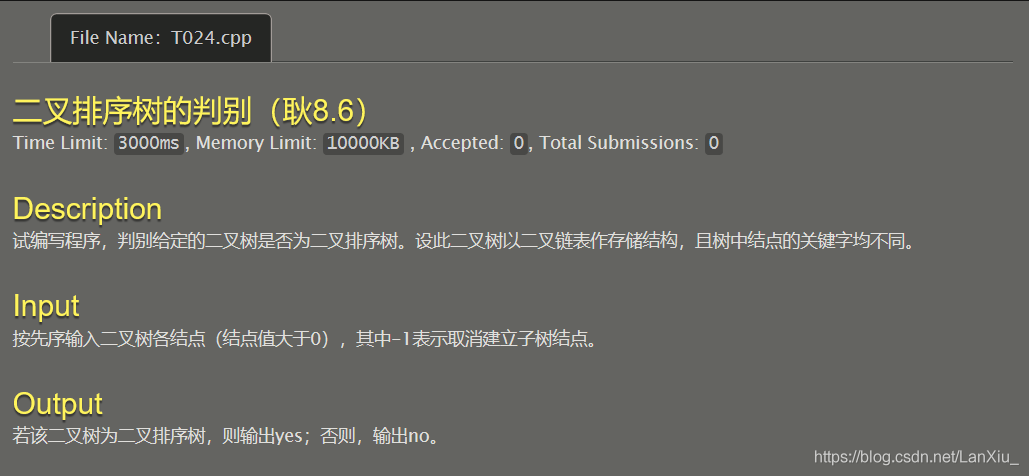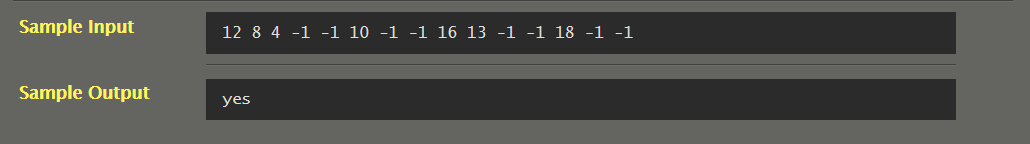#include<stdio.h>
#include<stdlib.h>

typedef struct BinTreeNode
{
int data;
struct BinTreeNode *Lchild;
struct BinTreeNode *Rchild;
}BinTreeNode,*BinTree;

void CreateBinTree(BinTreeNode **T)//建立二叉树
{
int elem;
scanf("%d",&elem);
if(elem==-1) *T=NULL;
else{
*T=(BinTreeNode*)malloc(sizeof(BinTreeNode));
(*T)->data=elem;
CreateBinTree(&((*T)->Lchild));
CreateBinTree(&((*T)->Rchild));
}
}

int SelectBinSortTree(BinTreeNode *T)//判断
{
if(T==0) return 1;
else if(T->Lchild&&T->Rchild)
{
if(T->data<T->Lchild->data||T->data>T->Rchild->data) return 0;
else return(SelectBinSortTree(T->Lchild)&&SelectBinSortTree(T->Rchild));
}
else if(T->Lchild)
{
if(T->data<T->Lchild->data) return 0;
else return(SelectBinSortTree(T->Lchild));
}
else if(T->Rchild)
{
if(T->data<T->Rchild->data) return 0;
else return(SelectBinSortTree(T->Rchild));
}
return 1;
}

void printOrder(BinTreeNode *T)
{
if(T==NULL)return;
printOrder(T->Lchild);
printf("%d ",T->data);
printOrder(T->Rchild);
}

int main()
{
BinTreeNode *T;

CreateBinTree(&T);
//printOrder(T);
if(SelectBinSortTree(T))
{
printf("yes");
}
else printf("no");
return 0;
}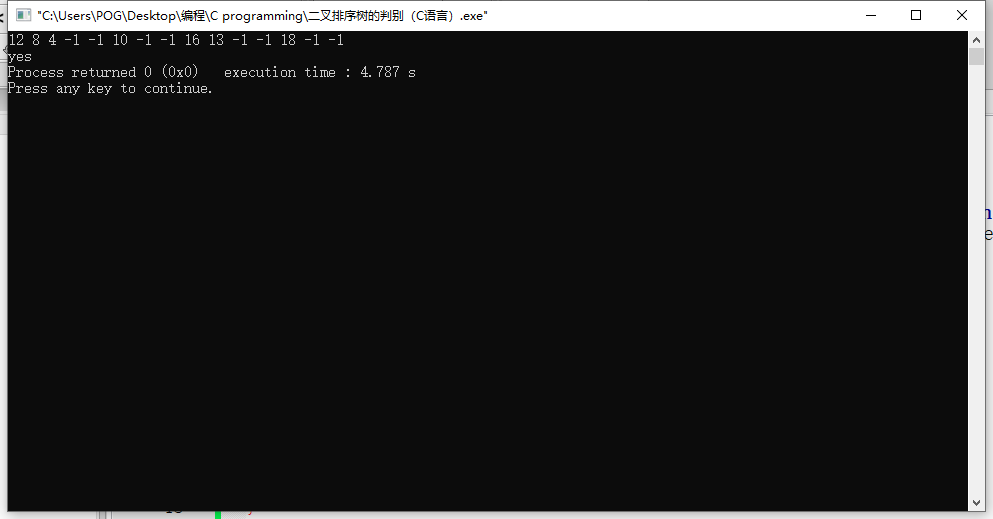我们中序输出：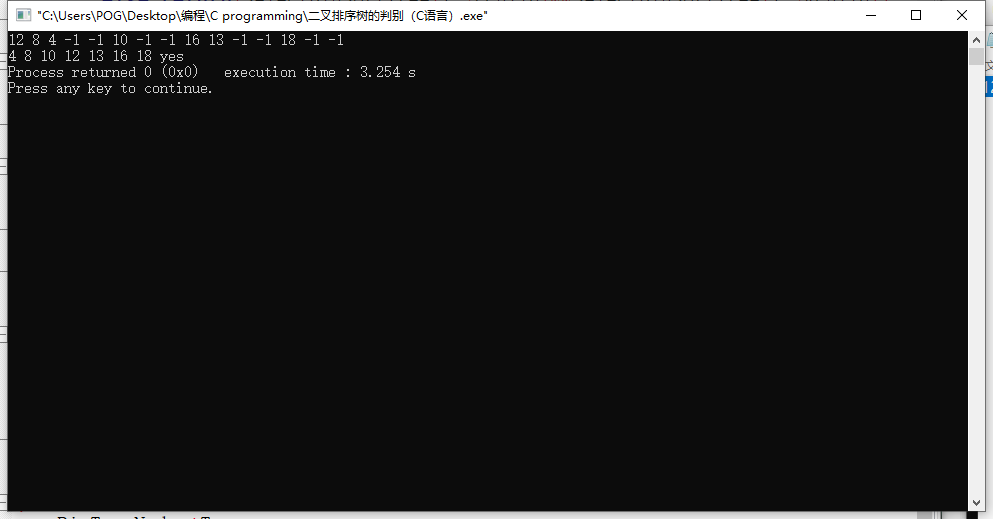可见为递增有序数列。

展开全文• 二叉排序树的中序序列递增有序序列。 对给定二叉树进行中序遍历，若前值比后值小，则为二叉排序树。 KeyType predt=-32767;//保存当前结点中序前驱的值 int JudgeBST(BiTree bt){ int b1,b2; if(bt==NULL)...
• 试写一个判别给定二叉树是否为二叉排序树的算法。以前序遍历序列和中序遍历序列给出该二叉树的结点，并创建该二叉树。然后再进行判断。请注意，树中结点关键字可能相同。 【样例输入1】 6 4 5 8 6 9 0 4 5 6 6 8 9 0...树结构 排序 数据结构
• 判断二叉排序树 采用二叉排序树的特征性质进行求解 中序遍历二叉排序树有序数组，递增，不存在相等的情况 bool isSortTree(Tree root){ //判断空 static int min =-1000; if(root==NULL){ return true; ...
• （3）左右子树分别一棵二叉排序树。 根据二叉排序树的定义，左子树结点值<根结点值<右子树结点值，则对二叉排序树进行中序遍历，可以得到一个递增的有序序列。如果有相同的值，可以将该节点放在左子节点或右...数据结构 二叉树 二叉排序树 java
• 一、二叉排序树概述 二叉排序树（Binary Sort Tree），又称二叉查找树（Binary Search Tree），亦称二叉搜索树。对于二叉排序树的任何一个非叶子节点，要求左子节点的值比当前节点的值小，右子节点的值比当前节点的...二叉排序树 二叉搜索树 二叉树 java 数据结构
• 《数据结构》实验报告班级:姓名：学号:E-mail:日期:◎实验题目: 建立二叉排序树...一、需求分析1、本程序中，输入一组数据，然后建立一棵二叉排序树存储这些元素，再中序遍历输出检验树建立是否正确，中序遍历输出的...
• 在分析treemap时，分析到了红黑树，而红黑树是由二叉排序树变体产生的，因此学习二叉排序树是常用树的基础；本篇文章主要分析二叉排序树的构建 ，及原理解析，遍历方式。数据结构 二叉树 java 算法
• 二叉排序树

2021-08-22 22:25:22
文章目录 二叉排序树 二叉排序树插入 查询 查找分析 插入 建立 二叉排序树删除    二叉排序树   二叉排序树： 或者是一棵空树；或者是具有下列性质的二叉树：(1)若它的左子树不空，则左子树上所有结点的值均小于...二叉树 数据结构 算法 二叉排序树
• int judgetree(BiTree T) { while(T){ if(T->lchild!=NULL&&T->lchild->data>T->data) return -1; if(T->rchild!=NULL&&T->rchild->... judgetree(...数据结构 c语言
• bool is_bst(int begin, int end, const vector& sequence) { cout |" ... } 传入一个元素类型int的数组，数组最后一位根节点，每次取到左右子树并递归是否为二叉搜索，得出该序列是否为一二叉搜索的后序遍历。数据结构
• 平衡二叉树是一种二叉排序树，其中每一个结点的左子树和右子树的高度差至多1 平衡因子BF（Balance Factor）=== 结点的左子树高度 −-− 结点的右子树高度 平衡二叉树上所有结点的BF只可能是{−1,0，1}\{-1,0，1\}{...数据结构 算法 平衡二叉树 二叉排序树
• 判断二叉树是否为二叉排序树 依据条件： 1.二叉排序树的中序遍历递增 （即使用中序遍历的递归结构） int pre = -256; int check = 1; //用于判断是否为排序树 int judge(BiTree T) { if(T->lchild&&...数据结构 c语言
• 二叉排序树（Binary Sort Tree），又称二叉查找树（Binary Search Tree），亦称二叉搜索树。是数据结构中的一类。在一般情况下，查询效率比链表结构要高。...（3）左、右子树也分别为二叉排序树； （4）没有键值相等...scala 数据结构 算法
• 标签：二叉树什么是二叉排序树二叉排序树或者是一颗空树，或者具有以下性质的二叉树：(1)若它的左子树不空，则左子树上的所有节点的值都小于他的父节点的值；(2)若它的右子树不空，则右子树上的所有节点的值都...
• 文章目录二叉排序树的定义二叉排序树的查找二叉排序树的插入二叉排序树的构造二叉排序树的删除 二叉排序树的定义 二叉排序树（Binary Sort Tree， BST），也称二叉查找树。 二叉排序树或者是一棵空树，或者是一棵...c++ 二叉排序树 算法
• 二叉排序树（也称二叉查找树）。 性质：左子树结点值 < 根结点值 < 右子树结点值 所以对二叉排序树进行中序遍历，可以得到一个递增的xu'l二叉树 二叉排序树
• 接下去的n行有n个序列，每个序列格式跟第一个序列一样，请判断这两个序列是否能组成同一颗二叉排序树。 输出格式 如果二叉排序树相同则输出YES，否则输出NO 样例输入 2 567432 543267 57634..数据结构 算法 c语言 树形DP
• 二叉排序树的删除情况比较复杂，有下面三种情况需要考虑。 1 删除叶子节点。(比如：2,5,9,12)。 2 删除只有一颗子树的节点。(比如：1)。 3 删除有两颗子树的节点.。(比如：7,3,10 )。 二 思路分析 对删除结点...算法
• 二叉查找 二叉树包含许多特殊的形式，每一种形式都有自己的作用，但是其最主要的应用还在于进行查找操作和维持相对顺序这两个方面。 二叉查找的定义（Binary Search Tree）： 该是一颗二叉树。 如果左子树...
• 二叉排序树的基本操作: 1、查找 2、增加 3、删除。 代码展示及结果截图: #include<stdio.h> #include<stdlib.h> /* 二叉排序树 <==> 二叉搜索树 (BST) <==> 二叉查找树 1. 二叉...
• 前几天完成数据结构课程设计，需要求二叉树ASL值，去网上查了查，发现都还要用栈... } void BSTaslplus(BSTree T) { cout 该二叉排序树ASL值：" (T, 0) * 1.0 / BSTnodecnt(T) ; return; } 如果有什么问题，欢迎指正数据结构 排序算法 c++ 算法
• 题目1201：二叉排序树时间限制：1 秒内存限制：32 兆特殊判题：否提交：6508解决：2747题目描述：输入一系列整数，建立二叉排序数，并进行前序，中序，后序遍历。输入：输入第一行包括一个整数n(1<=n<=100)。......